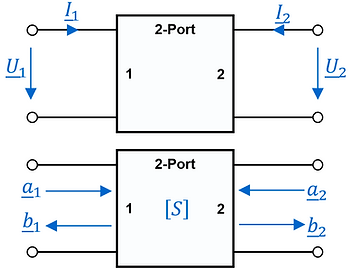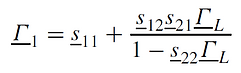top of page

This section introduces some of the most common Radio-Frequency (RF) parameters used in the field of EMC:

Scattering Parameters (S-Parameters).

Scattering Parameters} - also called S-parameters - are commonly used in high-frequency or microwave engineering to characterize a two-port circuit (see the picture below). The scattering parameters describe the relation of the power wave parts a1, b1, a2, and b2 that are transferred and reflected from a two-port's input and output. Generally speaking, the waves going towards the n-port are defined as a = (a1, a2, ..., an) and the waves traveling away from the n-port are defined as b = (b1, b2, ..., bn). The physical dimension for the incident a and reflected power waves is not Watt [W], it is the square root of watt [√W].Generally speaking, the S-parameter sij is determined by driving port j with an incident wave of voltage Vj+ and measuring the outgoing voltage wave Vi- at port i. Considering the picture above, the four scattering parameters can be computed as follows:where a1 [√W] is the incoming power wave at port 1 of a two-port, b1 [√W] is the outgoing power wave at port 1 of a two-port, a2 [√W] is the incoming power wave at port 2 of a two-port and b2 [√W] is the outgoing power wave at port 2 of the two-port.

The S-parameters are often given in [dB]:The forward traveling power towards port 1 P1fwd [W] and the reflected power from port 1 P1ref [W] can be written as:Of all four S-parameters, s11 and s21 are the most often used S-parameters in practice. s11 is often referred to as Return Loss (RL) or Reflection Coefficient Γ and s21 is often referred to as Insertion Loss (IL). More details about the terms RL and IL can be found in the sections below.

Due to the conservation of energy, we can write for a passive (non-amplifying) and lossless two-port (e.g., a lossless transmission line):For a passive and lossless two-port, the magnitude of |s11| can be calculated based on the magnitude of |s21| and vice-versa:Reflection Coefficient Γ.

We speak of matched impedances in case the load impedance Zload is the complex conjugate of the source impedance Zsource. In radiated emission and immunity EMC testing, it is important to understand the term matching and how to quantify it. All receiver and/or transmitter antennas must be matched to their receiver and/or transmitter equipment impedance (typical Z= 50Ω).The reflection coefficient Γ is defined as [4.4]:where Vreflected [V] is the reflected voltage waveform at a given point along the transmission line and Vforward [V] is the forward voltage waveform at the same point along the transmission line. A reflection coefficient Γ=0 means there is no reflection and a reflection coefficient of Γ=1 means there is total reflection. All variables are complex numbers. The reflection coefficient Γ is often given in [dB]:For a one-port, as shown in the picture above (with Zsource [Ω] and Zload [Ω]), the reflection coefficient Γ equals the scattering parameter s11. This means for a one-port the reflection coefficient Γ we can write:For a two-port with a matched impedance at port 2 (the load at the output matches the output impedance of the two-port, Γin the figure above is equal to 0), the reflection coefficient Γ1 at port 1 of the two-port is equal s11 of the two-port:However, for a two-port with an unmatched load at port 2 (ΓL≠0), a reflection happens at the output of the two-port, which influences the s11. This means that for a two-port with an unmatched output load on port 2, the input reflection coefficient Γ1 at port 1 is NOT equal s11. Instead, Γ1 it is [4.8]:VSWR.

VSWR means Voltage Standing Wave Ratio. The VSWR expresses the ratio of the maximum voltage Vmax [V] of a standing voltage wave pattern and the minimum voltage of a standing wave pattern Vmin [V] on a transmission line. A standing wave is a wave that oscillates in time but whose peak amplitude profile does not move along the transmission line (in the direction of z [m]).A VSWR value of 1.0 means perfectly matched. A VSWR value of infinity means a complete mismatch (100% of the forward wave is reflected). The VSWR can be calculated by using the reflection coefficient from above [4.5]:Return Loss [dB].

The Return Loss [dB] (RL) is the dB-value of the loss of power in the signal reflected (Preflected) by a discontinuity in a transmission line or due to an impedance mismatch. A low RL value indicates that not much power is transferred to the load and is reflected instead. Return loss [dB] is the negative value of the reflection coefficient Γ in [dB] [4.6].For a one-port load or a two-port with matched output load on port 2, the return loss RL can be calculated from the scattering parameter s11 like this:Insertion Loss [dB].

The term Insertion Loss (IL) is generally used for describing the amount of power loss due to the insertion of one or several of the following components (passive 2-port networks):

• Transmission Line (cable, PCB trace)

• Connector

• Passive FilterThe insertion loss (IL) represents the power ratio in [dB] of the power P1 and the power P2  of the picture above. P1 is the power, which would be transferred to the load in case the source is directly connected to the load. The power P2 represents the power that is transferred to the load in case the two-port network is inserted between the source and the load [4.2], [4.3].Insertion loss is often to be considered the same as the transmission parameter |s21|. However, the value of insertion loss and |s21| will be different if the input and output ports of a two-port aren't matched or use different reference impedances. Therefore, in the case that both ports of a two-port network use the same reference impedance and are matched, we can write:Free-Space Path Loss (FSPL).

The term free-space loss (FSL) or free-space path loss (FSPL) is important to understand for radiated emission and immunity testing. The FSPL is the attenuation of the electromagnetic field between a transmitter and a receiver antenna in free-space. It is assumed that the space between the antennas is free of obstacles and a line-of-sight path through free-space. As mentioned at the beginning of this chapter: all the formulas are valid for the far-field or in other words: free-space. In addition to that, the Friis transmission equation assumes matched impedances and matched polarization of the transmit and receiver antennas. The Friis transmission equation is stated as [4.7]:P= receiver antenna output power [W], P= transmitter antenna input power, Git = antenna gain of transmitter antenna, Gir = antenna gain of receiver antenna, λ = wave length [m] and = distance between transmitter and receiver antennas [m]. The free-space path loss FSPL is now defined as:= distance between transmitter and receiver antennas [m], λ = wave length [m], = speed of light 3E8[m/sec], = frequency [Hz].

For radiated EMC testing, the term link budget is often used. If you want to calculate the received power Pr [dBm] for a given receiver/transmitter setup (transmitter, frequency, antennas, distance, etc.), you calculate the link budget. In simple terms, this means:

Received Power Pr [dBm] = Transmitted Power [dBm] + Gains [dB] - Losses [dB].

If we rewrite the link budget formula above in a little more detailed way, we get:P= receiver antenna output power [dBm], P= transmitter antenna input power [dBm], L= transmitter losses (coaxial cable, connectors, etc.) [dB], Git = transmitter antenna gain [dB], FSPL = free-space path loss for a given frequency [dB], Lmisc = miscellaneous losses (fading, polarization mismatch, etc.) [dB], Gir = receiver antenna gain [dB], L= receiver losses (coaxial cable, connectors, etc.) [dB].

Impedance Matching Summary.

The table below shows how to convert between VSWR , return loss [dB] and the reflection coefficient . Z0 is the "system impedance" (typical Z= 50Ω or Z= 75Ω).In order to give you an idea what a good match means in terms of VSWR, reflection coefficient or return loss: we summarized all the values in this table below.Reflction Coefficint
VSWR
Return Loss
Insertion Loss
S-Parameters
Matching Summary